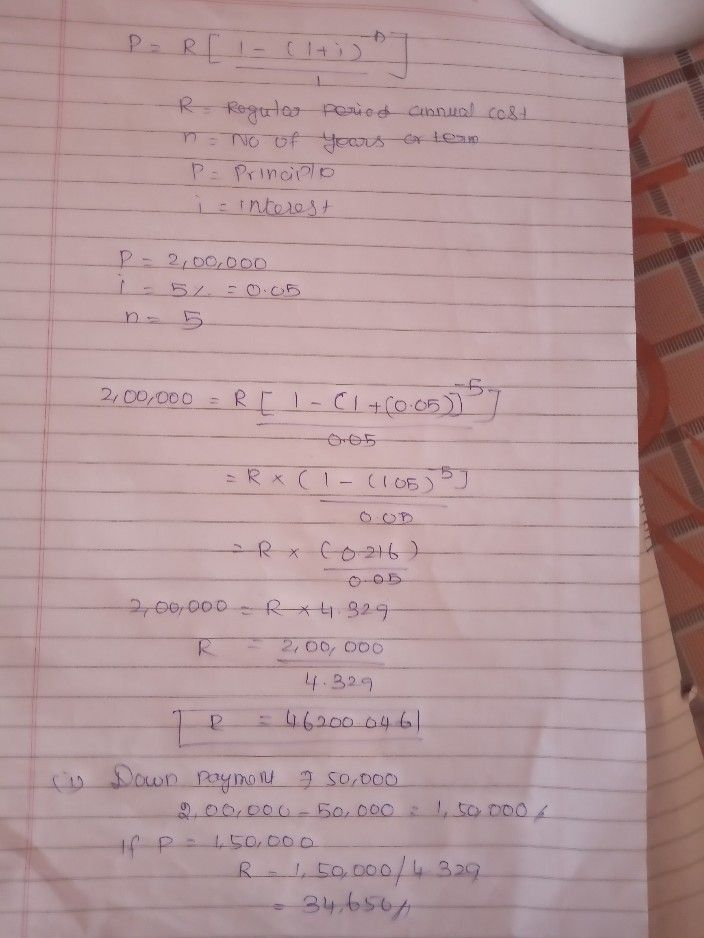Symbol
Problem$\bar{0.0414}$ = 5 years (app.) (0.5 Mr. X purchases a house for $\left(2,00.000.$ He agrees to pay for the house 5 equal instalments at the end of each year. If the money is worth 5% per annum effective, what would be the size of each instalment? In case X makes n down payment of 50,000, what would be size of each instalment? $\left(2012\right)$ Sol. (1) $P=Ra\bar{n} i=R \begin{cases} 1-\bar{\left(1+1\right)-n} \\ - \end{cases}$ $\left(mhcx$ $n=5:i=0.05:$ $P=82.00.000$ → $82.00.000=Ra$ 51.05 $2.00.000=R\left(4.326\right)$ $R=\dfrac {200000} {4.326}$ $=846r$ $232.09$ (ii) If down payment of $\left(50000$ is made then the amount to be paid in instalments $s=z\left(2.00,000-50,000\right)=$ $\left(1.50000$ If $P=81,50,000$ $R=$ $\dfrac {1.50000} {4.326}$ $=z34,674.06$ Q. 6. A machine depreciates each year by 10% of its value at the beginning valuo is 71 31 200. Find its origin
Other
SolutionQanda teacher - Elamathi P
soon will send solutionStudent
plzzz asap....
sir..Qanda teacher - Elamathi P
please waitsorry for the delay
if u have any questions let me know